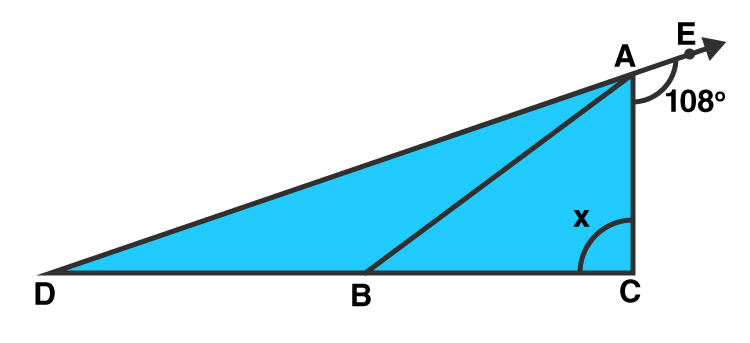# RD Sharma Solutions for Class 9 Maths Chapter 9 Triangle and its Angles Exercise 9.2

RD Sharma Solutions for Class 9 Maths Chapter 9 exercise 9.2 is available here. All questions in chapter 9 are prepared by subject experts to help students clear their doubts. RD Sharma Solutions Class 9 Exercise 9.2 is based on the basic concepts of exterior angles of a triangle, which states that, if a side of a triangle is produced, the exterior angle so formed is equal to the sum of the two interior opposite angles.

## Download PDF of RD Sharma Solutions for Class 9 Maths Chapter 9 Triangle and its Angles Exercise 9.2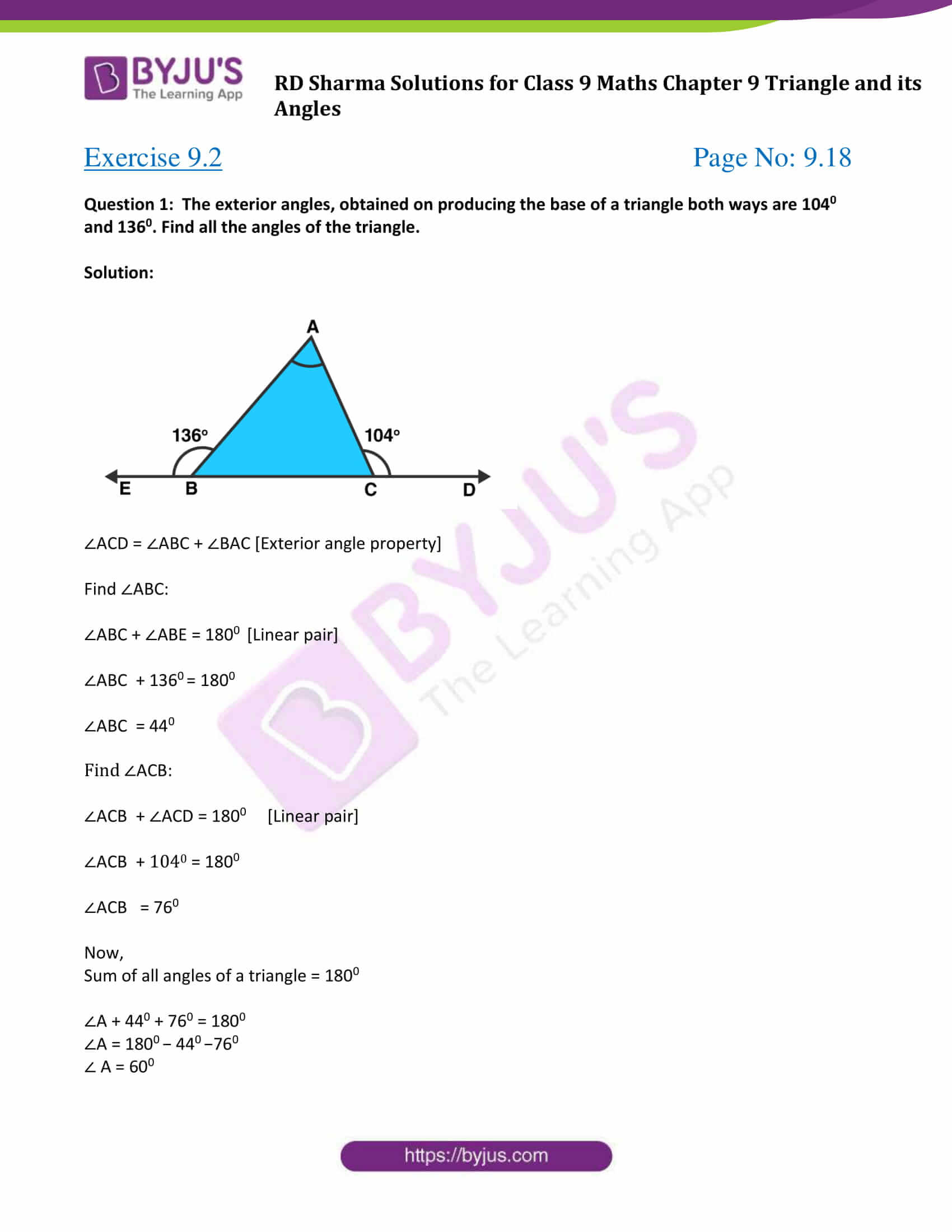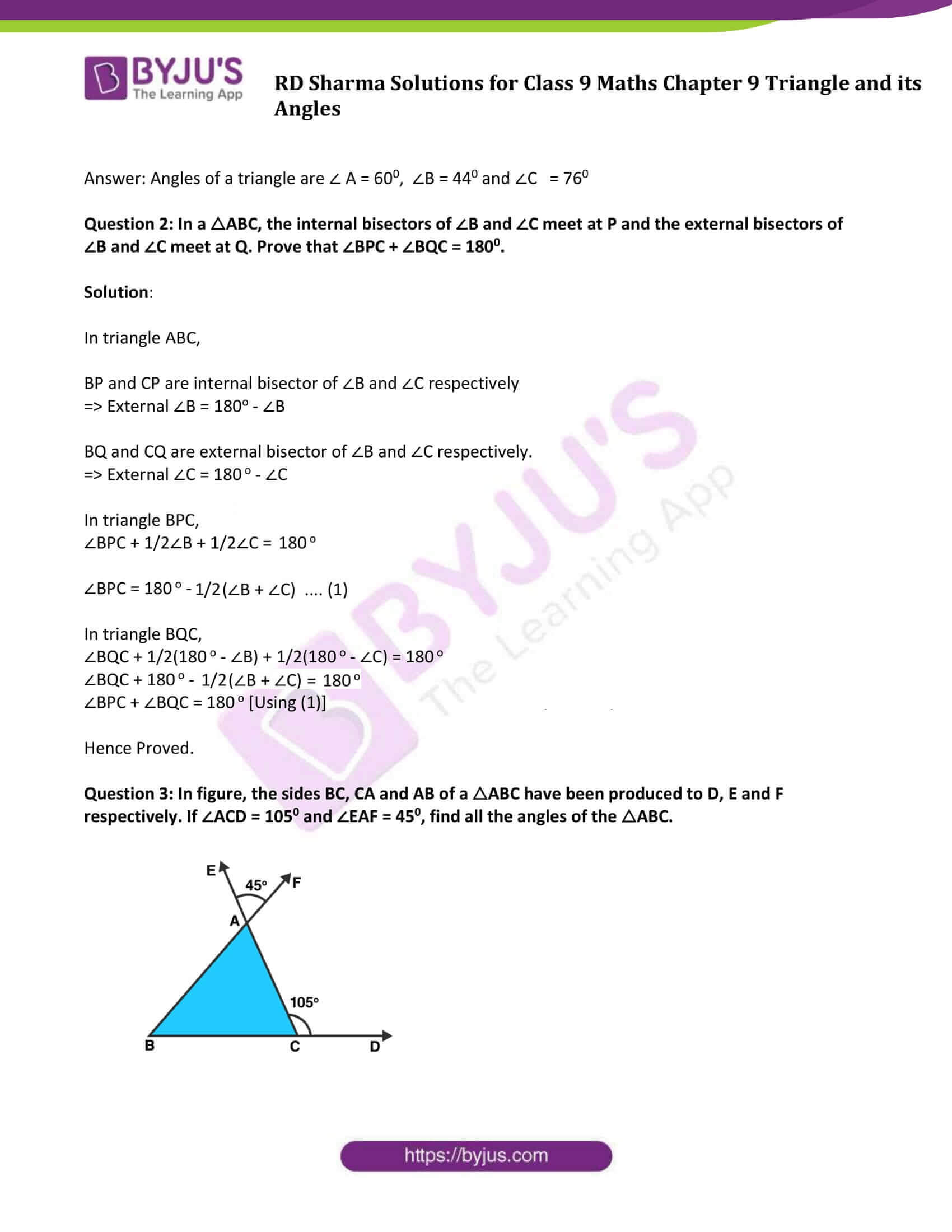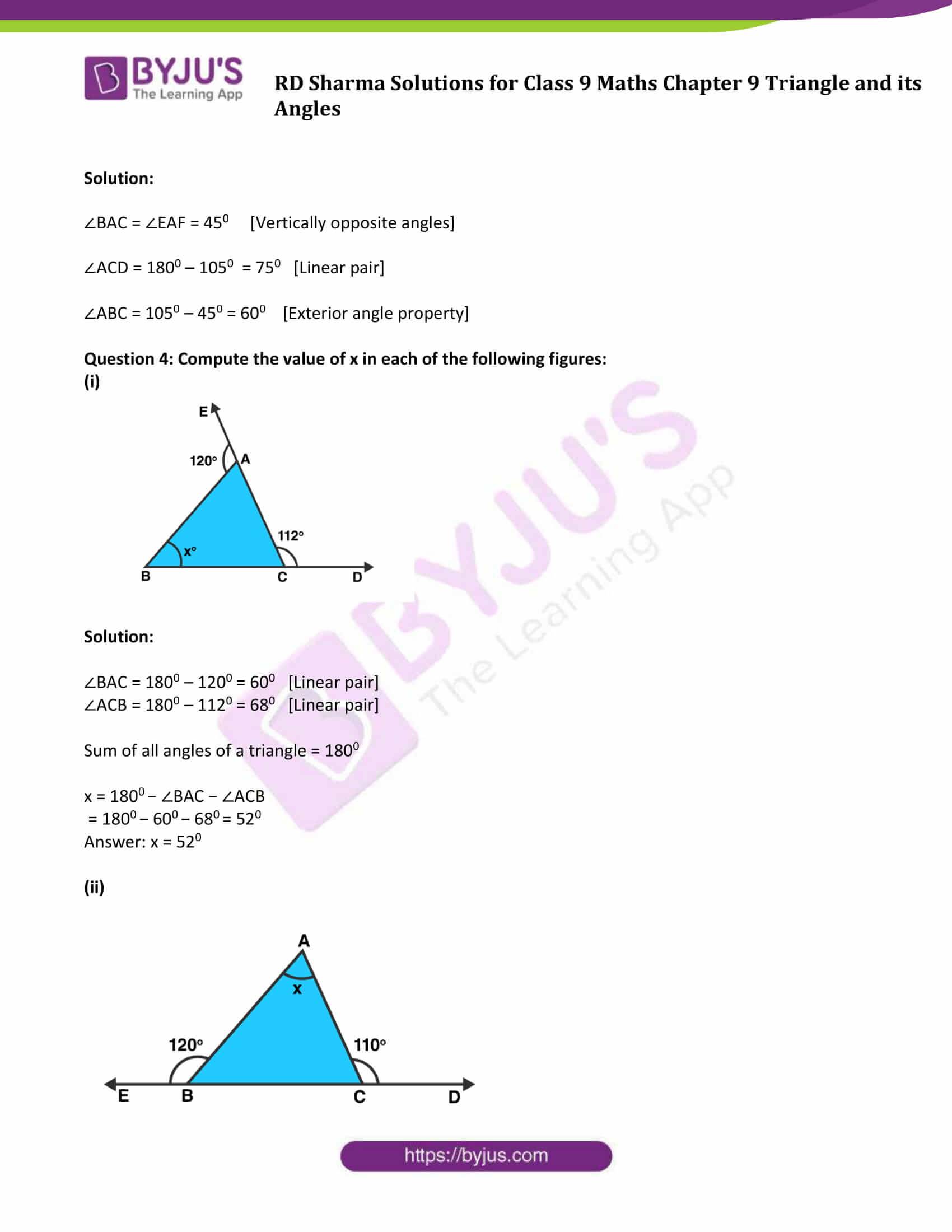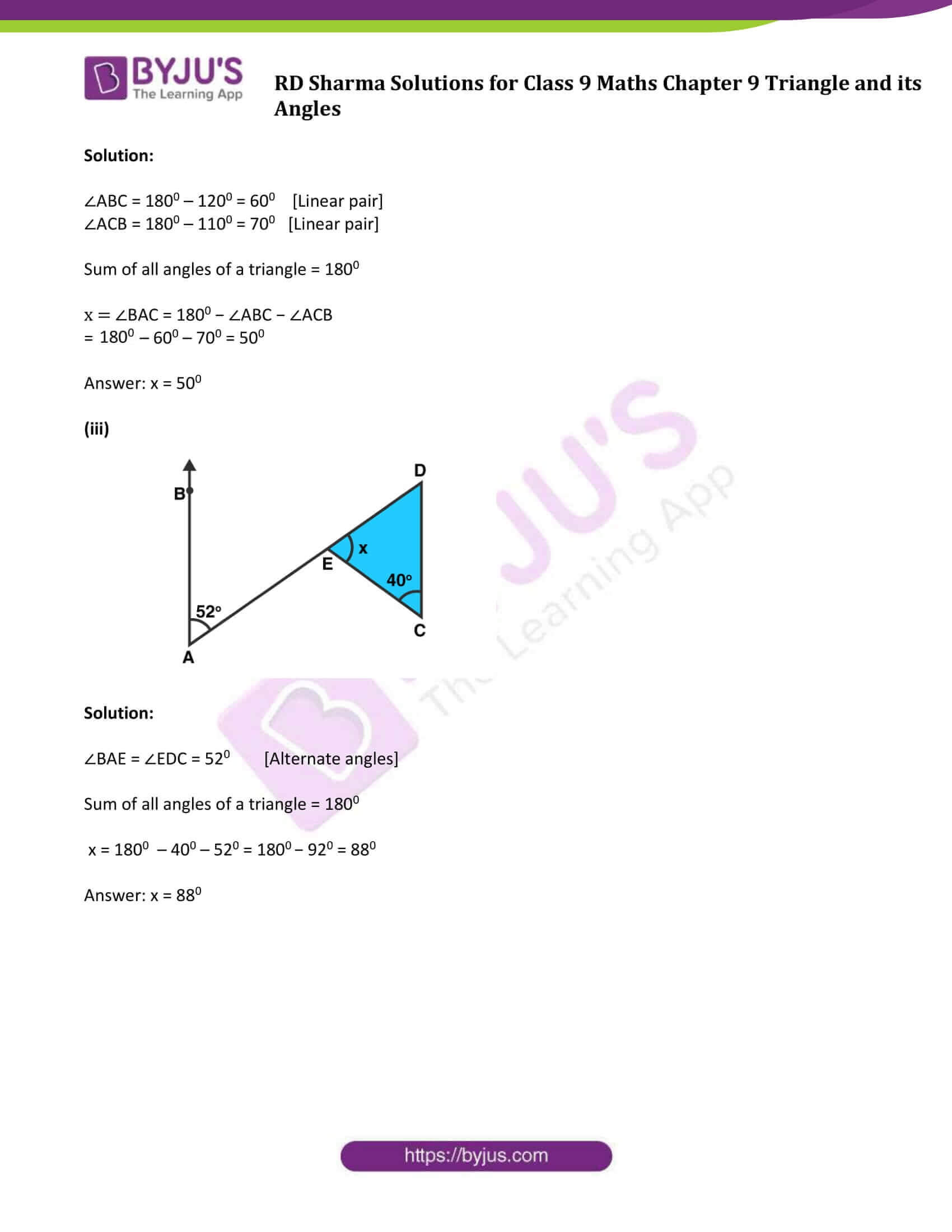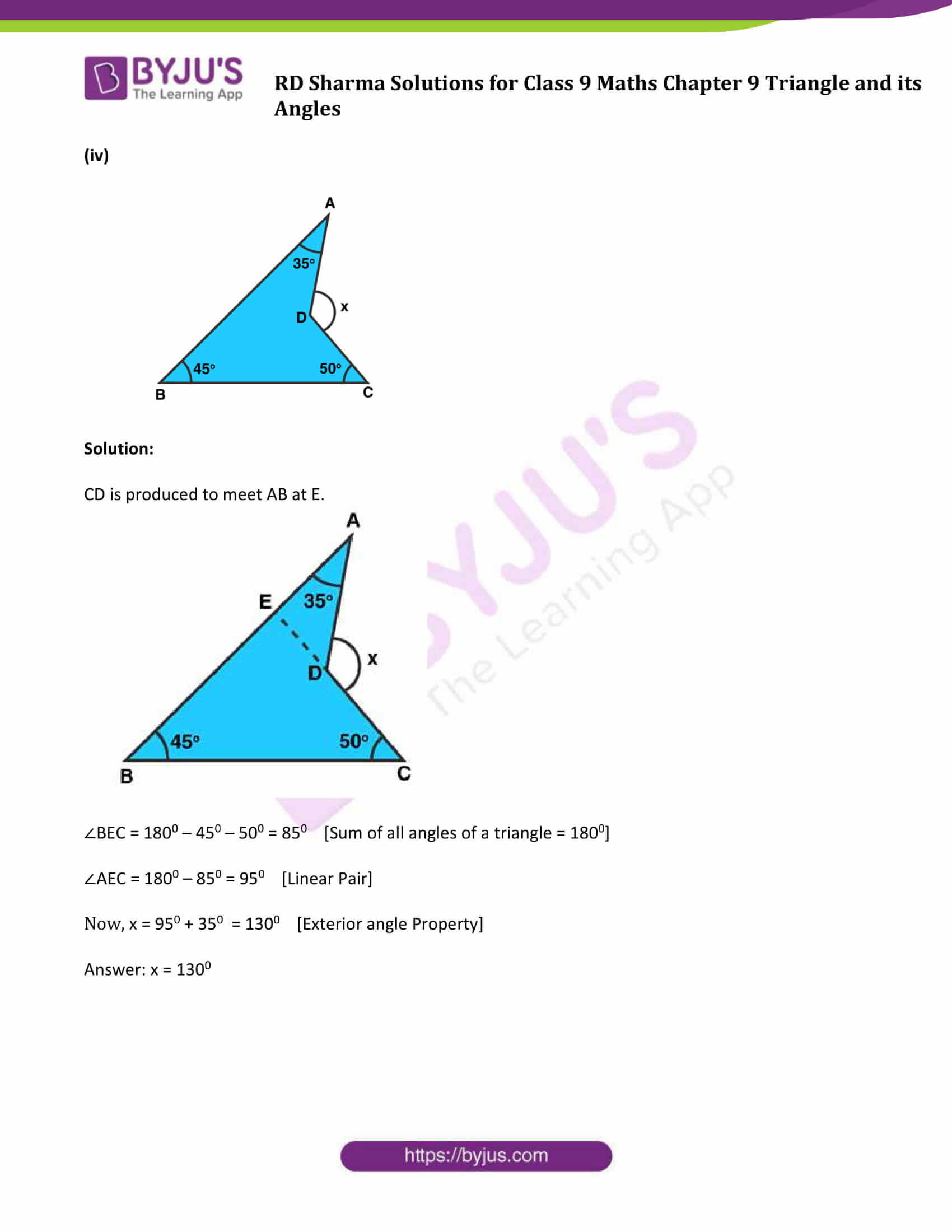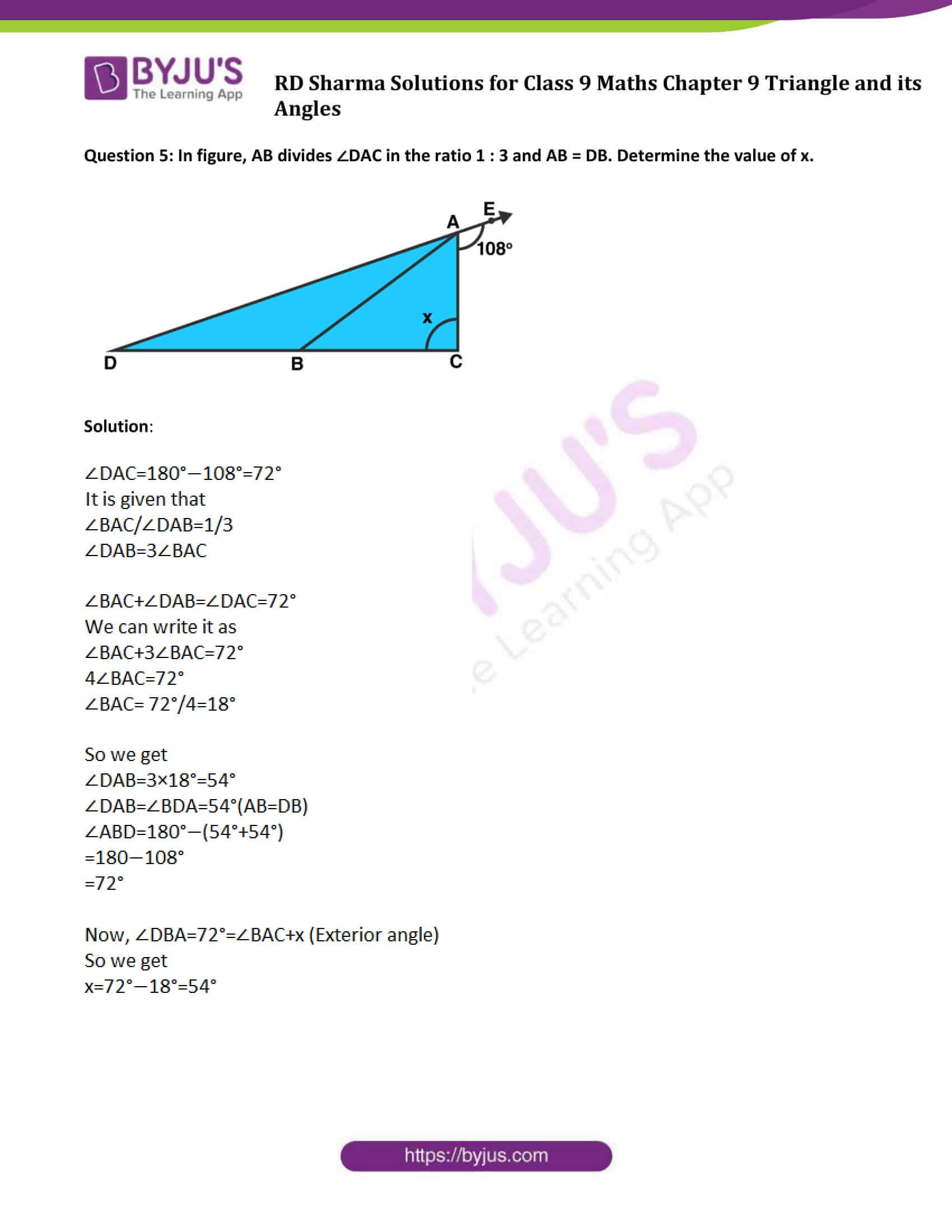### Access Answers to Maths RD Sharma Solutions for Class 9 Chapter 9 Triangle and its Angles Exercise 9.2 Page number 9.18

Question 1: The exterior angles, obtained on producing the base of a triangle both ways are 1040 and 1360. Find all the angles of the triangle.

Solution: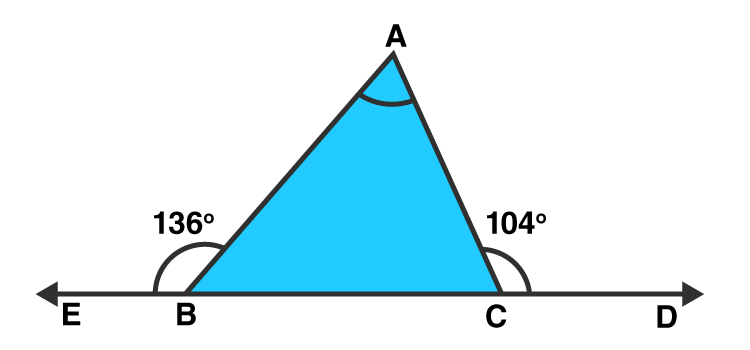∠ACD = ∠ABC + ∠BAC [Exterior angle property]

Find ∠ABC:

∠ABC + ∠ABE = 1800 [Linear pair]

∠ABC + 1360 = 1800

∠ABC = 440

Find ∠ACB:

∠ACB + ∠ACD = 1800 [Linear pair]

∠ACB + 1040 = 1800

∠ACB = 760

Now,

Sum of all angles of a triangle = 1800

∠A + 440 + 760 = 1800

∠A = 1800 − 440 −760

∠ A = 600

Answer: Angles of a triangle are ∠ A = 600, ∠B = 440 and ∠C = 760

Question 2: In a △ABC, the internal bisectors of ∠B and ∠C meet at P and the external bisectors of ∠B and ∠C meet at Q. Prove that ∠BPC + ∠BQC = 1800.

Solution:

In triangle ABC,

BP and CP are internal bisector of ∠B and ∠C respectively

=> External ∠B = 180o – ∠B

BQ and CQ are external bisector of ∠B and ∠C respectively.

=> External ∠C = 180 o – ∠C

In triangle BPC,

∠BPC + 1/2∠B + 1/2∠C = 180o

∠BPC = 180 o – 1/2(∠B + ∠C) …. (1)

In triangle BQC,

∠BQC + 1/2(180 o – ∠B) + 1/2(180 o – ∠C) = 180 o

∠BQC + 180 o – 1/2(∠B + ∠C) = 180 o

∠BPC + ∠BQC = 180 o [Using (1)]

Hence Proved.

Question 3: In figure, the sides BC, CA and AB of a △ABC have been produced to D, E and F respectively. If ∠ACD = 1050 and ∠EAF = 450, find all the angles of the △ABC.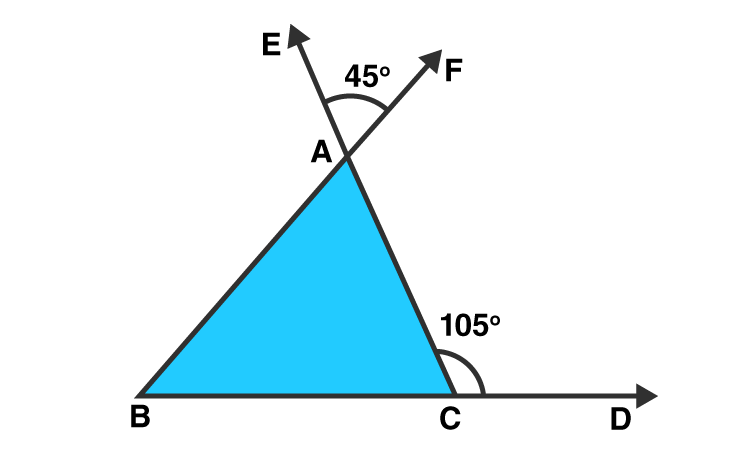Solution:

∠BAC = ∠EAF = 450 [Vertically opposite angles]

∠ACD = 1800 – 1050 = 750 [Linear pair]

∠ABC = 1050 – 450 = 600 [Exterior angle property]

Question 4: Compute the value of x in each of the following figures:

(i)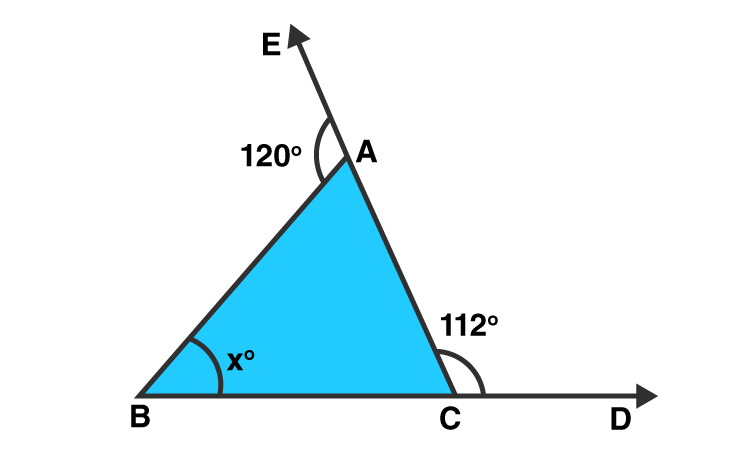Solution:

∠BAC = 1800 – 1200 = 600 [Linear pair]

∠ACB = 1800 – 1120 = 680 [Linear pair]

Sum of all angles of a triangle = 1800

x = 1800 − ∠BAC − ∠ACB

= 1800 − 600 − 680 = 520

(ii)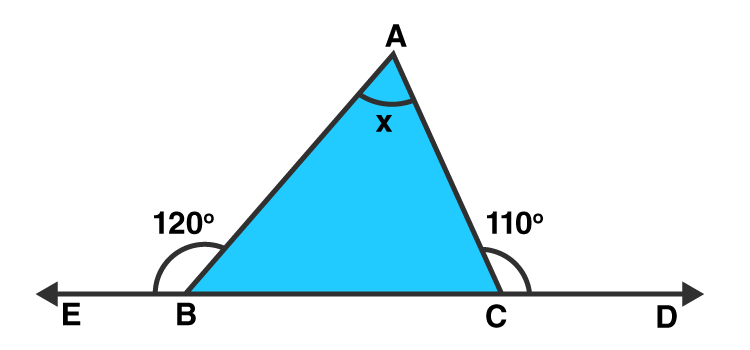Solution:

∠ABC = 1800 – 1200 = 600 [Linear pair]

∠ACB = 1800 – 1100 = 700 [Linear pair]

Sum of all angles of a triangle = 1800

x = ∠BAC = 1800 − ∠ABC − ∠ACB

= 1800 – 600 – 700 = 500

(iii)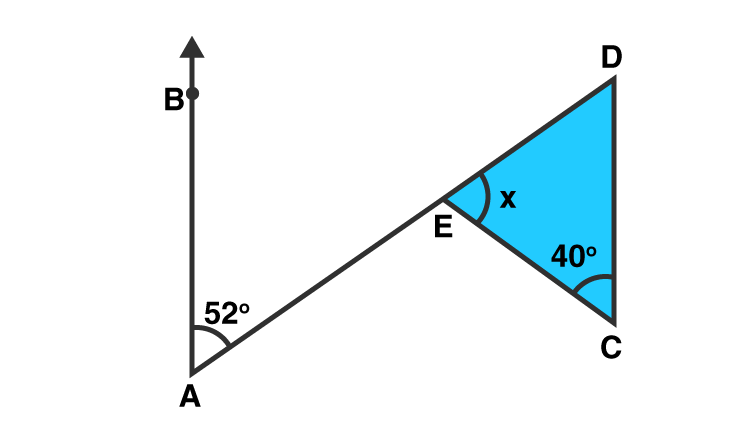Solution:

∠BAE = ∠EDC = 520 [Alternate angles]

Sum of all angles of a triangle = 1800

x = 1800 – 400 – 520 = 1800 − 920 = 880

(iv)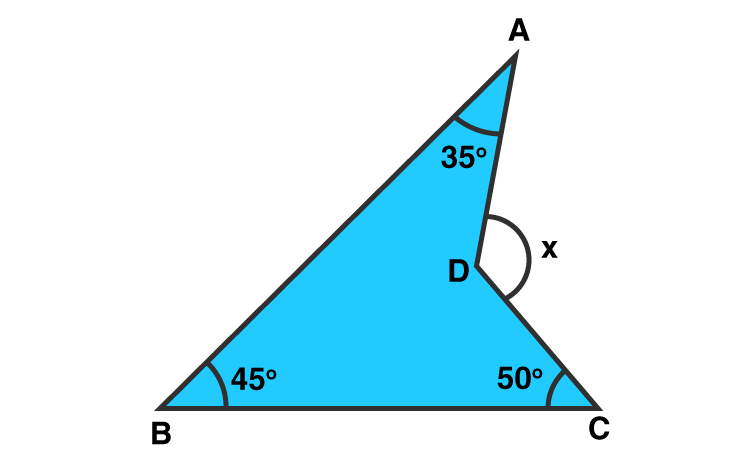Solution:

CD is produced to meet AB at E.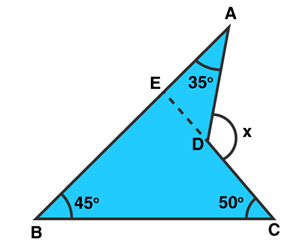∠BEC = 1800 – 450 – 500 = 850 [Sum of all angles of a triangle = 1800]

∠AEC = 1800 – 850 = 950 [Linear Pair]

Now, x = 950 + 350 = 1300 [Exterior angle Property]